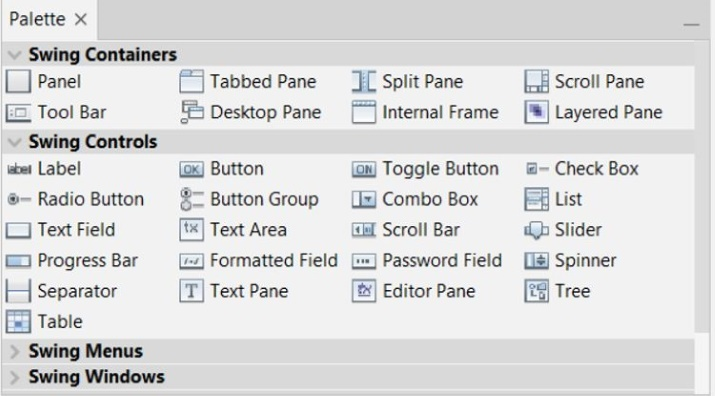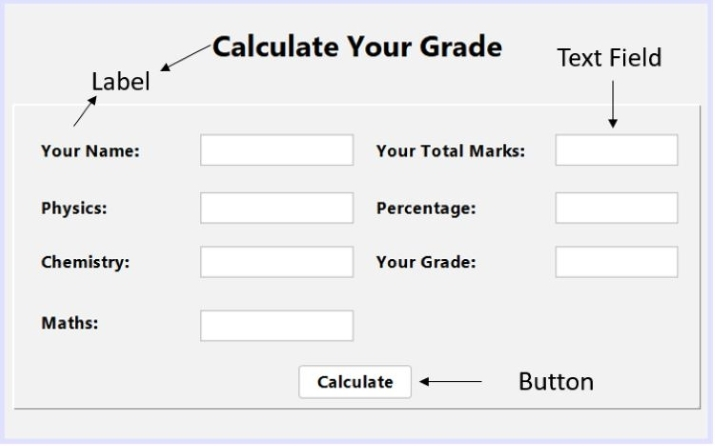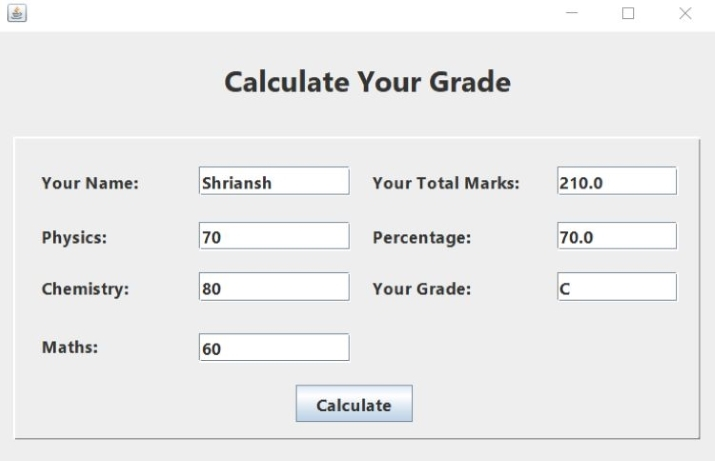# Student Grade Calculator using Java Swing

Swing is a framework of Java that is used to develop window based applications. It provides a rich variety of GUI (Graphical User Interface) components that are lightweight and platform independent. Some of the classes of GUI components are JLable, JButton, JFrame, JCheckBox and so forth.

In this article, we will build a simple student grade calculator using the components of Java Swing.

## Steps to create Student Grade Calculator using Java Swing

To develop this application follow these steps −

### Step 1

Open your Netbeans IDE and create a new Java Application through the following path − File -> New Project -> Java -> Java Application.

### Step 2

Go to your application folder and locate source package and then right click on default package -> New -> Jframe Form.JFrame Form will create an initial container where we can drag and drop components like Check Box, Text Field to make a full GUI.

The Swing provides following options to create GUI. We simply need to drag and drop to use these features.### Step 3

Using Label, Text Field and Button properties create this User Interface. It will accept marks for three subjects and when we click on Calculate button, the result will be visible in text fields of Total Marks, Percentage and Grade.### Step 4

At this step, we need to create code that will be generated automatically by Right clicking on UI and then go to properties -> code -> check the generate center and click close.

### Step 5

Once code gets generated, press Ctrl + f and Search for ‘UI’ and then replace ‘Nimbus’ with ‘Metal’.

### Step 6

Now, go to Design and double click on button ‘Calculate’. You will be redirected to the portion of source code where you can put your logic that ‘Calculate’ button is going to perform.

There copy and paste the following code.

### Example

private void jButton1ActionPerformed(java.awt.event.ActionEvent evt) {
double mh, ch, ph, sum, pr;
String grd;
// getting values of subjects entered by user
mh = Integer.parseInt(Sub3.getText());
ch = Integer.parseInt(Sub2.getText());
ph = Integer.parseInt(Sub1.getText());
sum = mh + ph + ch;
pr = (sum/300) * 100;
// calculating percentage
if(pr >= 90) {
grd = "A";
} else if(pr >= 80) {
grd = "B";
} else if(pr >= 70) {
grd = "C";
} else if(pr >= 60) {
grd = "D";
} else if(pr >= 50) {
grd = "E";
} else {
grd = "S";
}
// printing results in the text field of Marks, Percentage and Grade
Marks.setText(String.valueOf(sum));
Per.setText(String.valueOf(pr));
}


When we run the code, the below interface will be opened. There we need to enter the values in name and subjects section. Then, click ‘Calculate’ button to see the result. The result will be printed in the text field of ‘Marks’, ‘Percentage’ and ‘Grade’.

### Output## Conclusion

The swing is used to build Graphical User Interface where a user can interact and perform operations on it. It will enhance the user experience through its look and feel. In this article, we have developed a student grade calculator application where a student can find their grade by providing his/her marks.

Updated on: 12-May-2023

607 Views